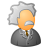# QlikView App Dev

Discussion Board for collaboration related to QlikView App Development.

Announcements
Our next Qlik Insider session will cover new key capabilities. Join us August 11th REGISTER TODAY
cancel
Showing results for
Did you mean:Not applicable

## Variable with set analysis and a string parameter

Hello,

I try to make a variable which contains a set analysis and a parameter in it.  After I put an expression in my chart with that variable but it does not work.

My variable vTest contains:   =Only({1<Code_Measures_et_Time={\$1}>} Time_Label)

In my chart I put this expression in the expression label : =\$(vTest(101_1001))

My parameter is a string : 101_1001

What am I doing wrong?

Thank you!

Patrick

1 Solution

Accepted SolutionsNot applicable
Author

My variable vTest must contains (i removed the equal sign

in my variable:   Only({1<Code_Measures_et_Time={\$1}>} Time_Label)

This way my variable doesn't recalculate before having my parameter I think.

In my chart I put this expression in the expression label :  =\$(vTest(101_1001))

But because I have a formula in my variable that need to be evaluated I added "=\$(" in front and ")" at end : =\$(=\$(vTest(101_1001)))

5 RepliesMVP

Maybe try

=Only({1<Code_Measures_et_Time={'\$1'}>} Time_Label)

(note the quotes). If you fill the variable with a LET statement, use chr(39) function calls to insert the quotes in a string.

Peter.Not applicable
Author

Thanks but I already tried that and it didn't help.Not applicable
Author

My variable vTest must contains (i removed the equal sign

in my variable:   Only({1<Code_Measures_et_Time={\$1}>} Time_Label)

This way my variable doesn't recalculate before having my parameter I think.

In my chart I put this expression in the expression label :  =\$(vTest(101_1001))

But because I have a formula in my variable that need to be evaluated I added "=\$(" in front and ")" at end : =\$(=\$(vTest(101_1001)))MVP

You're right, should have seen that. It's a common mistake that makes chart expressions fall over. Good deduction.Contributor III

Is there a way to put multiple string in a single parameter ?

For eg:

Expression:

Let vE_Sales = sum({<Year = {\$1}>} Sales);

When calling this expression we say

\$(vE_Sales('2016'))

But if I wanted to do the same for multiple years. Let say 2016 & 2015. how do we do pass multiple string values in a single parameter ?

\$(vE_Sales('2016', '2015'))Community Browser